# California State University Fresno Introduction to Data Structure

• Slides: 12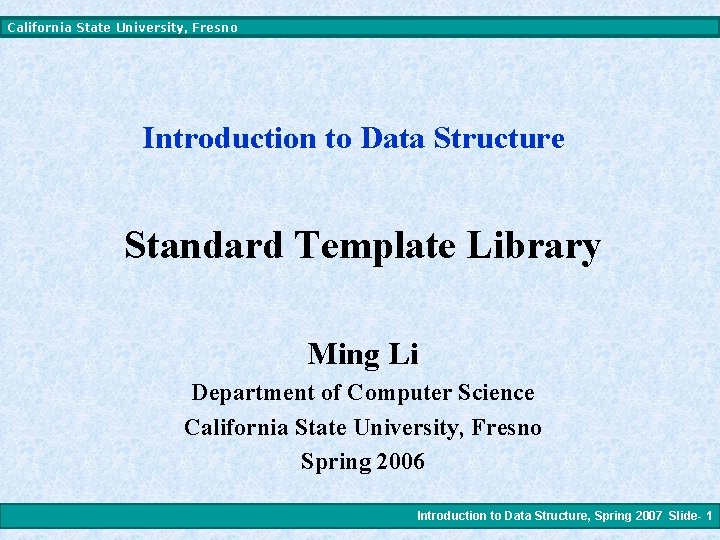California State University, Fresno Introduction to Data Structure Standard Template Library Ming Li Department of Computer Science California State University, Fresno Spring 2006 Introduction to Data Structure, Spring 2007 Slide- 1California State University, Fresno Template Syntax • • Template function syntax includes the keyword template followed by a non-empty list of formal types enclosed in angle brackets. In the argument list, each type is preceded by the keyword typename, and types are separated by commas. Format: template <typename T, typename U, typename V, . . . > Introduction to Data Structure, Spring 2007 Slide- 2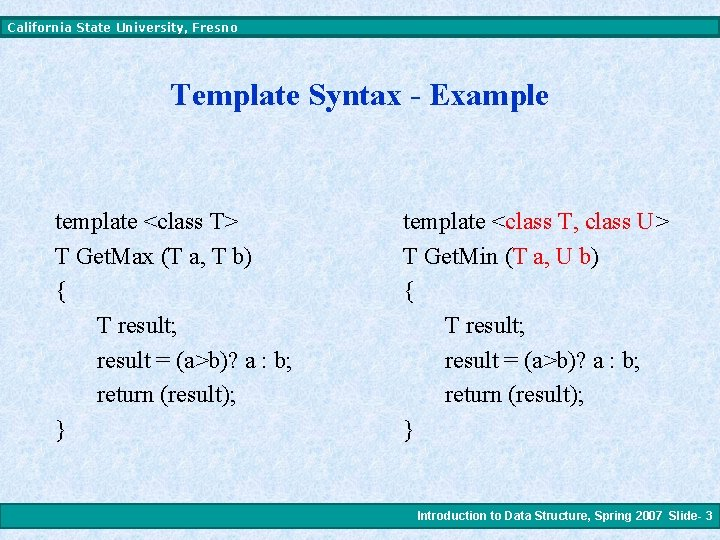California State University, Fresno Template Syntax - Example template <class T> T Get. Max (T a, T b) { T result; result = (a>b)? a : b; return (result); } template <class T, class U> T Get. Min (T a, U b) { T result; result = (a>b)? a : b; return (result); } Introduction to Data Structure, Spring 2007 Slide- 3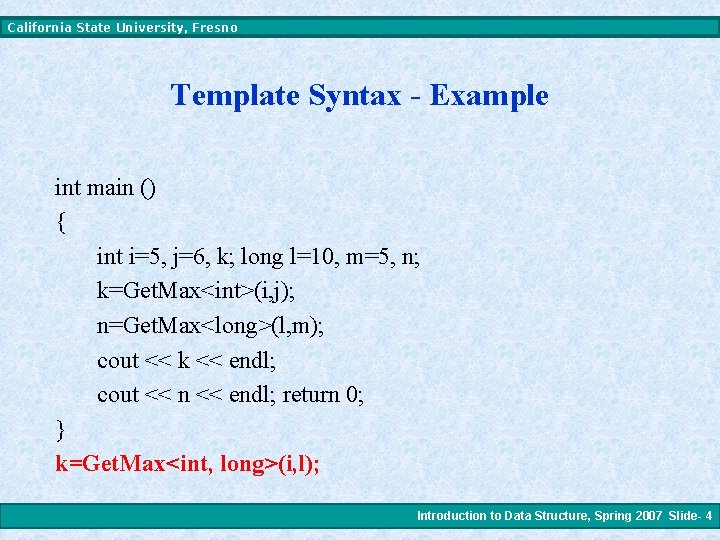California State University, Fresno Template Syntax - Example int main () { int i=5, j=6, k; long l=10, m=5, n; k=Get. Max<int>(i, j); n=Get. Max<long>(l, m); cout << k << endl; cout << n << endl; return 0; } k=Get. Max<int, long>(i, l); Introduction to Data Structure, Spring 2007 Slide- 4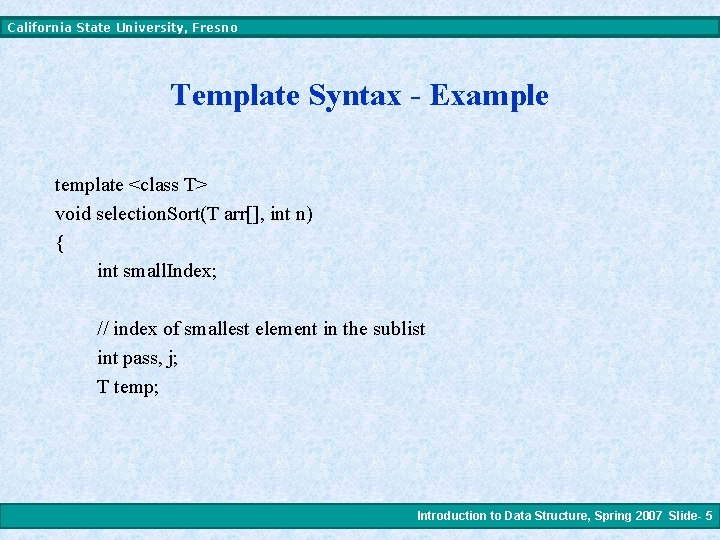California State University, Fresno Template Syntax - Example template <class T> void selection. Sort(T arr[], int n) { int small. Index; // index of smallest element in the sublist int pass, j; T temp; Introduction to Data Structure, Spring 2007 Slide- 5California State University, Fresno Template Syntax - Example for (pass = 0; pass < n-1; pass++) { small. Index = pass; for (j = pass+1; j < n; j++) if (arr[j] < arr[small. Index]) small. Index = j; if (small. Index != pass) { temp = arr[pass]; arr[pass] = arr[small. Index]; arr[small. Index] = temp; } } } How to use this template? ? ? Introduction to Data Structure, Spring 2007 Slide- 6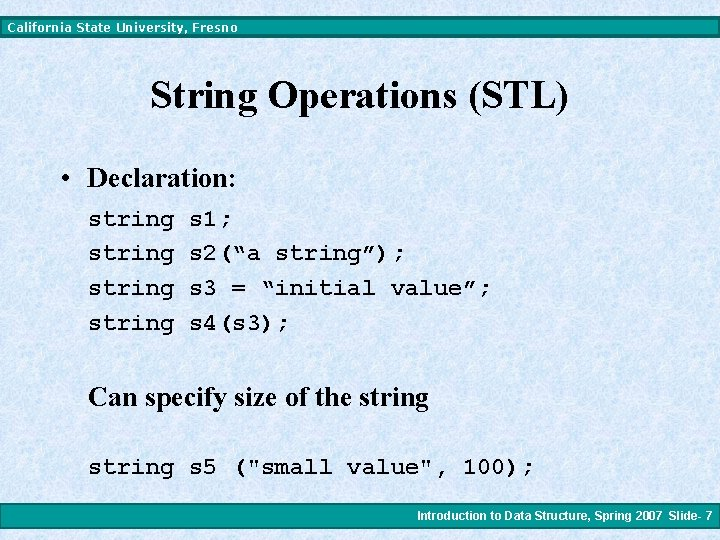California State University, Fresno String Operations (STL) • Declaration: string s 1; string s 2(“a string”); string s 3 = “initial value”; string s 4(s 3); Can specify size of the string s 5 ("small value", 100); Introduction to Data Structure, Spring 2007 Slide- 7California State University, Fresno String Operations (STL) • Getting size and capacity s 3. size() – returns number of characters s 3. length() – returns number of characters s 3. capacity() – returns the capacity of the string • Resetting size and capacity s 3. resize(50) – change the size to 50 s 3. reserve(200) – change the capacity to 200 • Checking the size s 3. empty() – Is s 3 empty? Introduction to Data Structure, Spring 2007 Slide- 8California State University, Fresno String Operations (STL) • Access s. c_str(); - return C_style string s. substr(start, len); – returns a substring c = s[i]; - return an element c = s. at(i); - return an element • Comparison if(s 1 == s 2) - return true if s 1 equals s 2 i = s. compare(s 2) – return <0 if s<s 1, 0 if s==s 1, or >0 if s>s 1 i = s. compare(start, len, s 1, start 1, len 1) compare two different strings Introduction to Data Structure, Spring 2007 Slide- 9California State University, Fresno String Operations (STL) • Altering s[i] = c; s. at(i) = c; s. append(s 1); - concatenate s 1 to s s. assign(s 1, start, len) – change content of s s. clear() – empty s s. erase(50, 14) – erase 14 characters after the 50 th character s. insert(10, s 1) – insert s 1 to s at index 10 s. replace(i 1, n 1, s 1, i 2, n 2) Introduction to Data Structure, Spring 2007 Slide- 10California State University, Fresno String Operations (STL) • Search i = s. find(c); Position of leftmost occurrence of char c. i = s. find(s 1); Position of leftmost occurrence of s 1. i = s. rfind(s 1); As find, but right to left. i = s. find_first_of(s 1); Position of first char in s which is in s 1 set of chars. i = s. find_first_not_of(s 1); Position of first char of s not in s 1 set of chars. i = s. find_last_of(s 1); Position of last char of s in s 1 set of chars. i = s. find_last_not_of(s 1); Position of last char of s not in s 1 set of chars. Introduction to Data Structure, Spring 2007 Slide- 11California State University, Fresno Reference • List of all functions of C++ Strings: http: //www. cppreference. com/cppstring/index. html • C-Style String functions: http: //www. cplus. com/ref/cstring/ Introduction to Data Structure, Spring 2007 Slide- 12